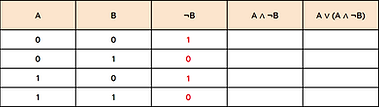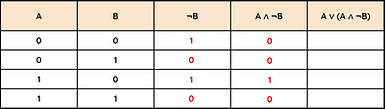top of page

## What is a logical operator?

Inside of each computer system are millions of transistors.

These are tiny switches that can either be turned on (represented in binary by the number 1) or turned off (represented by 0).

Logical operators are symbols used to represent circuits of transistors within a computer. The three most common operators are:

• NOT

• AND

• OR

## What is a truth table?

A truth table is a visual way of displaying all possible outcomes of a logical operator.

The input and output values in a truth table must be a Boolean value - usually 0 or 1 but occasionally True or False.

## OR

A NOT logical operator will produce an output which is the opposite of the input.

NOT is also known as Negation.

The symbol for NOT is ¬

An AND logical operator will output 1 only if both inputs are also 1.

AND is also known as Conjunction.

The symbol for AND is

An OR logical operator will output 1 if either input is 1.

OR is also known as Disjunction.

The symbol for OR is

## OR Logic Gate Symbol## Truth Table## Multiple Operators

Exam questions could ask you complete truth tables that use more than one logical operator.

Work out each column in turn from left to right and look carefully at which preceding column you need to use.NOT B

A AND NOT B

A OR (A AND NOT B)

## Logic Diagrams

You may be asked in an exam to draw a logic diagram when given a logical expression.

Draw any NOT symbols or expressions in brackets first.A logic diagram for C = ¬A ∧ BA logic diagram for D = C ∨ (A ∧ B)## Questo's Questions

4.1 - Boolean Logic:

1. Copy and complete the following truth tables:

1b. Simplify the expression in the second truth table.

2a. A cinema uses a computer system to monitor how many seats have been allocated for upcoming movies. If both the premium seats and the standard seats are sold out then the system will display a message. State the type of logical operator in this example.

2b. For the more popular movies, the cinema's computer system will also display a message if either the premium seats or the standard seats have exclusively been sold out. However, it will not output a message when both have been sold out. State the type of logical operator in this example.

3. Draw a logic diagram for C = (¬B v A) ∧ A.bottom of page